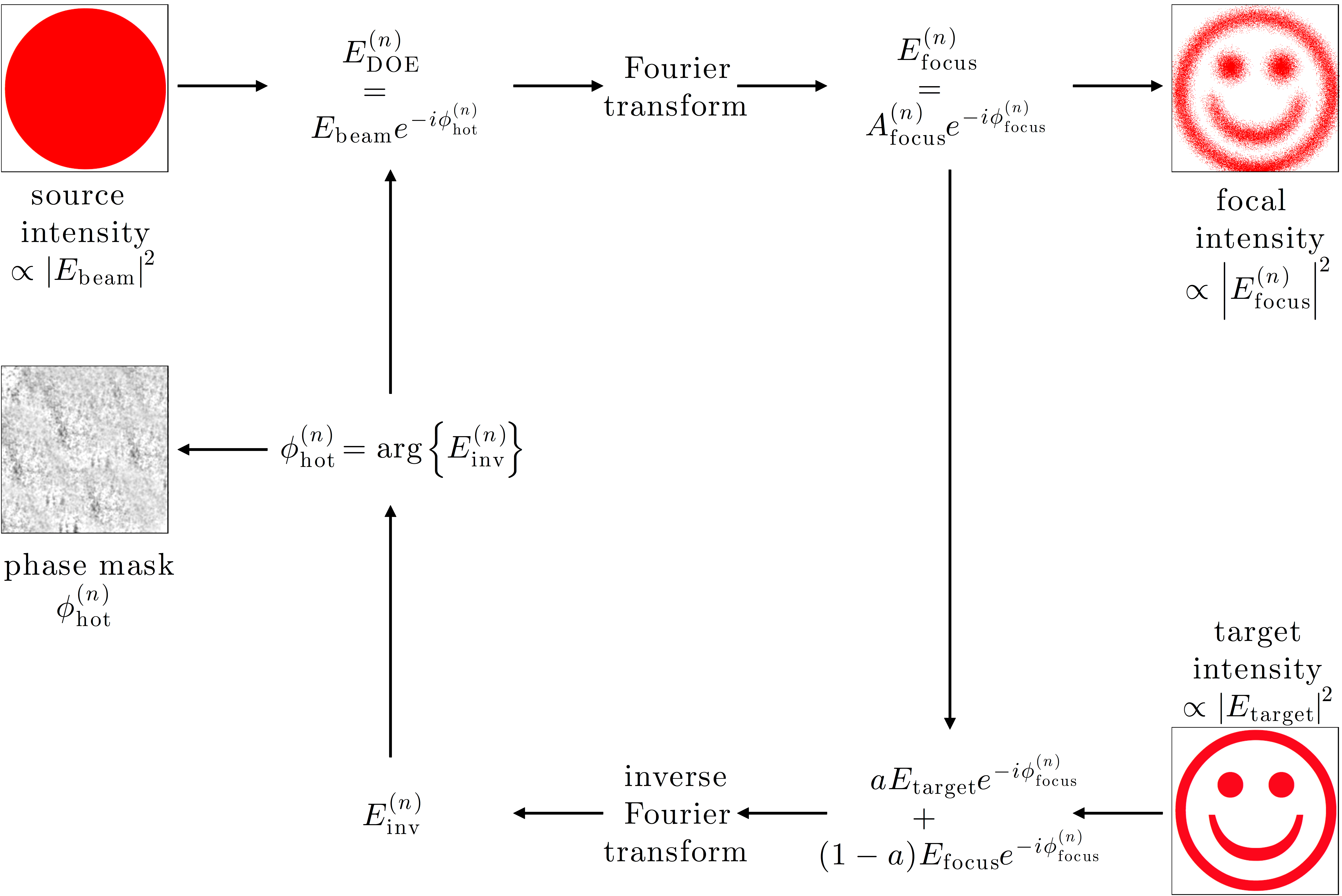# Figure 11.5 — The adaptive-additive algorithmFigure 11.5 — The adaptive-additive algorithm. The algorithm is quite similar to the Gerchberg–Saxton algorithm illustrated in Fig. 11.4. At the nth iteration, the amplitude of the field at the DOE, EDOE(n), is given by Ebeam and its phase by φhot(n). The focal field Efocus(n) is given by the Fourier transform of EDOE(n). At this point, the amplitude of the focal field is substituted with a weighted sum of the target amplitude and the focal field amplitude itself. The resulting field is inverse Fourier transformed, obtaining Einv(n) Finally, the hologram phase is updated to the phase of Einv(n). If the focal intensity and the target intensity differ more than an acceptable error, the cycle is then repeated (until a certain maximum number of iterations is reached).